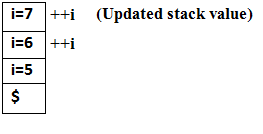Pre Increment and Post Increment concept in C programming is very important. Here we are discussing Unary operators with example and explanations so that you can easily understand the fundamentals of Pre increment and Post increment in C programming. So Let’s start with the basics first.

In the C programming Unary operators are having higher priority than the other operators rest of the Suffix/postfix increment and decrements, Function call, Array subscripting etc. operators.  Unary operators having widely scope in loop construction with increment or decrements a value by 1. It is very easy to use and understand C Programming operators. A complete detail about these unary operators of C language is given below with suitable easy code example.

There are two Unary Operators used in C Programming language;

1. Increment and
2. Decrements

Increment Unary Operator in C Programming:

An operator that takes current value of variable and adding one in them and provide resultant value.

Properties and features of Increment unary operator:

• An increment operator is used to increment the current value of variable by adding integer 1.
• An increment operator can be applied to only variables.
• An increment operator is denoted by ++ (double addition symbol continuously).

Types of Increment operator is used in C programming:

• Pre-increment and
• Post-increment

Pre-increment unary operator:

Pre-increment unary operator is used to increment the value of variable by one before using in the expression. In the Pre-Increment operator concern value is first incremented and then it used inside the expression with final updated value.

Syntax of Pre-increment unary operator:

• ++variable;

Example Pre-increment unary operator:

• ++i; //it is equivalents to the i=i+1; or i+=1 ;

Programming Code 1:

#include <stdio.h>
int main()
{
int i=5;
printf(“%d\n”,++i);
return 0;
}

Output: 6

Explanation: In the above example the value of ‘i’ is initialize by 5 after that applying the pre increment operation on variable ‘i’ then it becomes 6 and the output is printed as 6.

In other words, it is explain as if we push the expression in to the stack the value of variable ‘i’ in pre-increment could not be execute until the expression completely execute. After completely execution of expression in stack the value of variable ‘i’ is incremented by 1 and the output of the expression is 6 because pre-increment unary operator uses the final updated value of the stack i.e. top value in the stack.Programming Code 2:

#include <stdio.h>
int main()
{
int i=5;
printf(“%d , %d\n”,++i ,++i);
return 0;
}

Output: 7 , 7

Explanation: In the above example the value of ‘i’ is initialize by 5 after that applying the pre-increment operation on variable ‘i’ then it becomes 6 and ones again applying pre-increment operator it becomes i=7, the output is printed as 7 , 7 (because after complete execution of the expression the value of variable ‘i’ is 7, So both the pre-incremented variable updated at last with updated value of variable ‘i’).

In other words, it is explain by expression execution in stack operation. We push the expression in to the stack the value of variable ‘i’ in pre-increment and could not be executing until the expression completely execute. After completely execution of expression in the stack the value of variable ‘i’ is incremented by 1 twice and the output of the expression is 7 , 7 because pre-increment unary operator uses the final updated value of the stack i.e. top value in the stack.

Post-increment unary operator:

In Post-increment first of all the loop executes then value of the concern variable is increments by 1 and return the value before present update state.

Post-increment unary operator is used to increment the value of variable as soon as after executing expression completely in which post increment is used individually. In the Post-Increment, value is first used in a expression and then incremented. In other words Post-increment unary operator returns the value before present state updation.

Syntax of Post-increment unary operator:

• variable++;

Example Post-increment unary operator:

• ++i;

Programming Code 1:

#include <stdio.h>
int main()
{
int i=5;
printf(“%d\n”,i++);
return 0;
}

Output: 5

Explanation: In the above example the value of ‘i’ is initialize by 5 after that applying the post increment operation on variable ‘i’ then it updated 6 but the output is print before update i.e. 5.

Programming Code 2:

#include <stdio.h>
int main()
{
int i=5;
printf(“%d , %d\n”,i++ , i++);
return 0;
}

Output: 6 , 5

Note: The execution of Unary Operator (Post-increment or Pre-increments execute form Right to left).

Hope this will be useful for you – But It’s not just End Here is a Quiz on this Pre Increment and Post Increment in C programming – Attempt Now

1 COMMENT

1.Rupesh Kumar

Thank You ,sir I read continuesly and we understand…
I hope that you understand mine easy to Way……

This site uses Akismet to reduce spam. Learn how your comment data is processed.